Impact Factor 4.677 | CiteScore 5.4
More on impact ›

# Frontiers in Neuroscience## ORIGINAL RESEARCH article

Front. Neurosci., 04 April 2018 | https://doi.org/10.3389/fnins.2018.00210

# Stochastic IMT (Insulator-Metal-Transition) Neurons: An Interplay of Thermal and Threshold Noise at Bifurcation

• 1School of Electrical and Computer Engineering, Georgia Institute of Technology, Atlanta, GA, United States
• 2Department of Electrical Engineering, University of Notre Dame, Notre Dame, IN, United States

Artificial neural networks can harness stochasticity in multiple ways to enable a vast class of computationally powerful models. Boltzmann machines and other stochastic neural networks have been shown to outperform their deterministic counterparts by allowing dynamical systems to escape local energy minima. Electronic implementation of such stochastic networks is currently limited to addition of algorithmic noise to digital machines which is inherently inefficient; albeit recent efforts to harness physical noise in devices for stochasticity have shown promise. To succeed in fabricating electronic neuromorphic networks we need experimental evidence of devices with measurable and controllable stochasticity which is complemented with the development of reliable statistical models of such observed stochasticity. Current research literature has sparse evidence of the former and a complete lack of the latter. This motivates the current article where we demonstrate a stochastic neuron using an insulator-metal-transition (IMT) device, based on electrically induced phase-transition, in series with a tunable resistance. We show that an IMT neuron has dynamics similar to a piecewise linear FitzHugh-Nagumo (FHN) neuron and incorporates all characteristics of a spiking neuron in the device phenomena. We experimentally demonstrate spontaneous stochastic spiking along with electrically controllable firing probabilities using Vanadium Dioxide (VO2) based IMT neurons which show a sigmoid-like transfer function. The stochastic spiking is explained by two noise sources - thermal noise and threshold fluctuations, which act as precursors of bifurcation. As such, the IMT neuron is modeled as an Ornstein-Uhlenbeck (OU) process with a fluctuating boundary resulting in transfer curves that closely match experiments. The moments of interspike intervals are calculated analytically by extending the first-passage-time (FPT) models for Ornstein-Uhlenbeck (OU) process to include a fluctuating boundary. We find that the coefficient of variation of interspike intervals depend on the relative proportion of thermal and threshold noise, where threshold noise is the dominant source in the current experimental demonstrations. As one of the first comprehensive studies of a stochastic neuron hardware and its statistical properties, this article would enable efficient implementation of a large class of neuro-mimetic networks and algorithms.

## 1. Introduction

A growing need for efficient machine-learning in autonomous systems coupled with an interest in solving computationally hard optimization problems has led to active research in stochastic models of computing. Optimization techniques (Haykin, 2009) including Stochastic Sampling Machines (SSM), Simulated Annealing, Stochastic Gradients etc., are examples of such models. All these algorithms are currently implemented using digital hardware which first creates a mathematically accurate platform for computing, and later adds digital noise at the algorithm level. Hence, it is enticing to construct hardware primitives that can harness the already existing physical sources of noise to create a stochastic computing platform. The principal challenge with such efforts is the lack of stable or reproducible distributions, or functions of distributions, of physical noise. One basic stochastic unit which enables a systematic construction of stochastic hardware has long been known—the stochastic neuron (Gerstner and Kistler, 2002)—which is also believed to be the unit of computation in the human brain. Moreover, recent studies (Buesing et al., 2011) have demonstrated practical applications like sampling using networks of such stochastic spiking neurons. There have been some attempts for building neuron hardware (Indiveri et al., 2006; Pickett et al., 2013; Mehonic and Kenyon, 2016; Sengupta et al., 2016; Tuma et al., 2016), but building a neuron with self-sustained spikes, or oscillations, which are stochastic in nature and where the probability of firing is controllable using a signal has been challenging. Here, we demonstrate and analytically study a true stochastic neuron (Jerry et al., 2017a) which is fabricated using oscillators (Shukla et al., 2014a,b; Parihar et al., 2015) based on insulator-metal transition (IMT) materials, e.g., Vanadium Dioxide (VO2), wherein the inherent physical noise in the dynamics is used to implement stochasticity. The firing probability, and not just the deterministic frequency of oscillations or spikes, is controllable using an electrical signal. We also show that such an IMT neuron has similar dynamics as a piecewise linear FitzHugh-Nagumo (FHN) neuron with thermal noise along with threshold fluctuations as precursors of bifurcation resulting in a sigmoid-like transfer function for the neural firing rates. By analyzing the variance of interspike interval, we determine that for the range of thermal noise present in our experimental demonstrations, threshold fluctuations are responsible for most of the stochasticity compared to thermal noise.

## 2. Materials and Methods

### 2.1. IMT Phase Change Neuron Model

A stochastic IMT neuron is fabricated using relaxation oscillators (Shukla et al., 2014b; Parihar et al., 2015) composed of an IMT phase change device, e.g., Vanadium Dioxide (VO2), in series with a tunable resistance, e.g., transistor (Shukla et al., 2014a) (Figure 1A). An IMT device is a two terminal device with two resistive states—insulating (I) and metallic (M), and the device transitions between the two states based on the applied electric field (which in turn changes the current through the device and the corresponding temperature) across it. The phase transitions are hysteretic in nature, which means that the IMT (insulator-to-metal) transition does not occur at the same voltage as the MIT (metal-to-insulator) transition. For a range of values of the series resistance, the resultant circuit shows spontaneous oscillations due to hysteresis and a lack of stable point (Parihar et al., 2015). Overall, the series resistance acts as a parameter for bifurcation between a spiking (or oscillating) state and a resting state of an IMT neuron.

FIGURE 1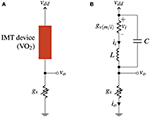Figure 1. (A) VO2 based IMT spiking neuron circuit consisting of a VO2 device in series with a tunable resistance. (B) Equivalent circuit of IMT neuron using a series inductance L and a parallel capacitance C.

The equivalent circuit model for an IMT oscillator is shown in Figure 1B with the hysteretic switching conductance gv(m/i) (gvm in metallic and gvi in insulating state), a series inductance L, and a parallel internal capacitance C. Let the IMT and MIT thresholds of the device be denoted by vh and vl, respectively, with vh > vl, and the current-voltage relationship of the hysteretic conductance be

$vi=h(ii,s)$

where h is linear in ii and s is the state—metallic (M) or insulating (I).

The system dynamics is then given by:

with ii and vo as shown in Figure 1B and s is considered as an independent variable.

### 2.2. Mechanism of Oscillations and Spikes

In VO2, IMT, and MIT transitions are orders of magnitude faster than RC time constants for oscillations, as observed in frequency (Kar et al., 2013) and time-domain measurements for voltage driven (Jerry et al., 2016) and photoinduced transitions (Cocker et al., 2012). As such, the change in resistance of the IMT device is assumed to be instantaneous. Figure 2A shows the phase space ii × (vddvo). V-I curves for IMT device in the two states metallic (M) and insulating (I) and the load line for series conductance vo = ii/gs for the steady state are shown along with the fixed points of the system S1 and S2 in insulating and metallic states respectively. The load line and V-I curves are essentially the nullclines of vo and ii, respectively. The capacitance- inductance pair delays the transitions and slowly pulls the system toward the fixed points S1 and S2 even when the IMT device transitions instantaneously. For small L/C ratio, the eigenvector (of the coefficient matrix) with large negative eigenvalue becomes parallel to the x-axis, whereas the other eigenvector becomes parallel to AB′ or BA′ depending on the state (M or I). When the system approaches A from below (or B from above) and IMT device is insulating (or metallic) with fixed point S1 (or S2), the IMT device transitions into metallic (or insulating) state changing the fixed point to S2 (or S1). Two trajectories are shown starting from points A and B each for the system (Equation 1)—one for small L/C value (solid) and the other for large L/C value (dashed). After a transition, the system moves parallel to x-axis almost instantaneously and spends most of the time following the V-I curve toward the fixed point. Before the fixed point is reached the MIT (or IMT) transition threshold is encountered which switches the fixed point, and the cycle continues resulting in sustained oscillations or spike generation.

FIGURE 2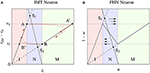Figure 2. (A) Trajectories (red) of system (1) in the phase space ii × (vddvo) for a small L/C value (solid) and a large L/C value (dashed). The ii-nullclines of system (1) are shown as solid black lines in the metallic (AB') and insulating (BA') states of the IMT device, and S1S2 is the vo-nullcline. Depending on the state, the phase space is divided into three vertical regions - I, M and N. In the region N the ii-nullclines are dependent on s (B) Nullclines of the FHN model in the phase space u × (1 − w) where f(u) is a piecewise linear function. The dynamics of FHN neuron are equivalent to the IMT neuron in the regions M and I. In the region N, for small L/C, the difference is only in the velocity and not the direction of system trajectories as they are parallel to x-axis.

### 2.3. Model Approximations and Connections With FHN Neuron

#### 2.3.1. Non-hysteretic Approximation

The model of (Equation 1) is very similar to a piecewise linear caricature of FitzHugh-Nagumo (FHN) neuron model (Gerstner and Kistler, 2002), also called the McKean's caricature (McKean, 1970; Tonnelier, 2003). Mathematically, the FHN model is given by:

where f(u) is a polynomial of third degree, e.g., f(u) = uu3/3, and Iext is the parameter for bifurcation, as opposed to gs in Equation (1). In the FHN model, one variable (u), possessing cubic nonlinearity, allows regenerative self-excitation via a positive feedback, and the second, a recovery variable (w), possessing linear dynamics, provides a slower negative feedback. It was reasoned in McKean (1970) that the essential features of FHN model are retained in a “caricature” where the cubic non-linearity is replaced by a piecewise linear function f(u). Nullclines of (Equation 2) with a piecewise linear f(u) are shown in Figure 2B in the phase space u × (1 − w). A function f(u) is trivially possible such that it is equal to vddh(ii, s) in the regions M and I, hence making the u-nullcline similar to the ii-nullcline in those regions. In the region N, the difference between f(u) and vddh(ii, s) for any state s does not result in a difference in the direction of system trajectories but only in their velocity, because for small L/C the trajectories are almost parallel to x-axis. Bifurcation in VO2 neuron is achieved by tuning the load line using a tunable resistance (gs), or a series transistor (Figure 3A). Figure 3B shows two load line curves corresponding to different gate voltages (vgs), where one gives rise to spikes while the other results in a resting state.

FIGURE 3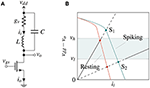Figure 3. (A) IMT neuron with series transistor used to achieve bifurcation between a spiking and a resting state. (B) Nullclines of the system with series transistor in the phase space ii × vddvo for two different vgs values for spiking and resting states. Bifurcation occurs when a stable points crosses the boundary of region vddvo ∈ [vl, vh].

#### 2.3.2. Single Dimensional Approximation

Moreover, a single dimensional piecewise approximation of the system can be performed using a dimensionality reduction by replacing the movement along the eigenvector parallel to the x-axis with an instantaneous transition from A to A′, or B to B′. This leaves a 1-dimensional subsystem in M and I each along the V-I curves AB′ and BA′. Experiments using VO2 show that the metallic state conductance gvm is very high which causes the charging cycle of vo to be almost instantaneous (Figure 4) and resembles a spike of a biological neuron. As such, the spiking statistics can be studied by modeling just the discharge cycle of vo. The inductance being negligible can be effectively removed and only the capacitance is needed for modeling the 1D subsystem of insulating state (Figure 6A) making vi = vddvo.

FIGURE 4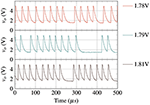Figure 4. Experimental waveforms of VO2 based spiking neuron for various vgs values (1.78, 1.79, and 1.81 V). A VO2 neuron shows almost instantaneous charging (spike) in metallic state.

### 2.4. Noise Induced Stochastic Behavior

The two important noise sources which induce stochasticity in an IMT neuron are (a) VIMT (vh) fluctuations (Zhang et al., 2016; Jerry et al., 2017b), and (b) thermal noise. Thermal noise η(t) is modeled in the circuit (Figure 6A) as a white noise voltage η(t)dt = σtdwt where wt is the standard weiner process and ${\sigma }_{t}^{2}$ is the infinitesimal thermal noise variance. The threshold vh is assumed constant during a spike, but varies from one spike to another. The distribution of vh from spike to spike is assumed to be Gaussian or subGaussian whose parameters are estimated from experimental observations of oscillations. If the series transistor always remains in saturation and show linear voltage-current relationship, as is the case in our VO2 based experiments, the discharge phase can be described by an Ornstein-Uhlenbeck (OU) process

where μ, θ, and σ are functions of circuit parameters of the series transistor, the IMT device and σt. The interspike interval is thus the first-passage-time (FPT) of this OU process, but with a fluctuating boundary.

#### 2.4.1. OU Process With Constant Boundary

Analytical expressions for the FPT of OU process (with μ = 0) for a constant boundary were derived using the Laplace transform method in Ricciardi and Sato (1988). Reproducing some of its results, let the first passage time for the system (Equation 3), with μ = 0, which starts at x(0) = x0 and hits a boundary S, be denoted by the random variable tf(S, x0), and its mth moment by τm(S, x0). Also, let $\stackrel{˜}{{t}_{f}}\left(S,{x}_{0}\right)$ be the FPT for another OU process with μ = 0, θ = 1, and σ = 2, and $\stackrel{~}{{\tau }_{m}}\left(S,{x}_{0}\right)$ be its mth moment. Then time and space scaling for the OU process imply that

where $\alpha =\sqrt{\frac{2}{\theta {\sigma }^{2}}}$. The first two moments for the base case OU process $\stackrel{~}{{\tau }_{1}}$ and $\stackrel{~}{{\tau }_{2}}$ are given by

where ϕk(z) can be written as an infinite sum

with ρ(n, k) being a function of the digamma function (Ricciardi and Sato, 1988).

#### 2.4.2. OU Process With Fluctuating Boundary

We extend this framework for calculating the FPT statistics with a fluctuating boundary S as follows. Let the IMT threshold be represented by the random variable vh. For the VO2 based IMT neuron, the 1D subsystem in the insulating phase can be converted in the form of Equation(3) with μ = 0 by translating the origin to the fixed point. If this transformation is T then x = Tvi = T(vddvo), S = Tvh, and xo = Tvl. The start and end points are B′ and A, respectively in Figure 2. vh is assumed constant during a spike, and across spikes the distribution of vh is vh ~ ${D}$, where ${D}$ is either Gaussian, or subGaussian. For subGaussian distributions we use the Exponential Power family EP[κ], κ being the shape factor. Let the interspike interval of IMT neuron be denoted by the marginal random variable ${t}_{imt}\left({D},{v}_{l}\right)$. Then timt is related to tf in Equation (4), given common parameters θ and σ, as follows:

$timt(D,vl)|(vh=v)=dtf(Tv,Tvl)$

The moments of timt can be calculated as:

where $\alpha =\sqrt{\frac{2}{\theta {\sigma }^{2}}}$. If ${D}$ is Gaussian or EP[κ] distribution and αT is an affine transformation, then αTvh also has a Gaussian or EP[κ] distribution.

### 2.5. Experiments

IMT devices are fabricated on a 10nm VO2 thin film grown by reactive oxide molecular beam epitaxy on (001) TiO2 substrate using a Veeco Gen10 system (Tashman et al., 2014). Planar two terminal structures are formed by patterning contacts using standard electron beam lithography which defines the device length (LVO2). Pd (20 nm)/Au (60 nm) contacts are then deposited by electron beam evaporation and liftoff. The devices are then isolated and the widths (WVO2) are defined using a CF4 based dry etch.

The IMT neuron is constructed using an externally connected n-channel MOSFET (ALD110802) and the fabricated VO2 device. A prototypical I-V curve is shown in Figure 5A. Within the experimental data, the current is limited to an arbitrarily chosen 200 μA to prevent a thermal runaway and breakdown of the device while in the low resistance metallic state. It should be noted that as the metallic state corresponds to the abrupt charging cycle of vo, limiting the current would not have noticeable effect on spiking statistics of the neuron.

FIGURE 5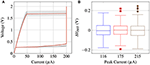Figure 5. (A) The prototypical DC voltage-current characteristics for a single VO2 device exhibits abrupt threshold switching at VIMT and VMIT. The current in the metallic state has been arbitrarily limited to a 200μA compliance current. (B) VIMT distribution as a function of the peak current during oscillations (value is set by the MOSFET saturation current). VIMT is extracted from 300+ cycles.

Threshold voltage fluctuations (cycle to cycle) were observed in all devices which were tested (>10). Threshold voltage distribution was estimated using the varying cycle-to-cycle threshold voltages collected from a single device. Thermal noise is not measured directly, but is estimated approximately by matching the simulation waveforms from the circuit model (Figure 6A) with the observed experimental waveforms. It can be verified that thermal noise of the transistor is not the dominant noise source by measuring the threshold variation as a function of the transistor current (Figure 5B) and observing that the distribution of switching threshold does not change with varying transistor current. Finally, the firing rate and its variation with vgs (Figure 6B) were measured for a single device.

FIGURE 6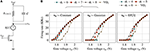Figure 6. (A) Noise model of IMT neuron where the noise components are the thermal noise voltage source η(t) and the IMT threshold fluctuation. (B) Firing rate plotted against vgs using the analytical model for different vh distributions (Constant, Gaussian, and EP) and comparison with experimental observations.

## 3. Results

### 3.1. Spiking Statistics

#### 3.1.1. First Moment and the Firing Rate

First moment of timt is calculated using Equations (5) and (7) as

$𝔼[timt(D,vl)]=θ(𝔼vh[ϕ1(αTvh)]−ϕ1(αx0))$

The expansion for ϕk(z) in Equation(6) can be used to calculate 𝔼vhkTvh)] using the moments of αTvh as follows

$𝔼vh[ϕk(αTvh)]=12k∑n=1∞(2)n𝔼[(αTvh)n]Γ(n2)ρ(n,k)n!$

Figure 6B shows firing rate ($1/𝔼\left[{t}_{imt}\left(D,{v}_{l}\right)\right]$) as a function of vgs for various σt values and for three distributions of threshold fluctuations. The calculations approximate the experimental observations well for all three vh distributions, the closest being EP with σt = 4.

#### 3.1.2. Higher Moments

For higher moments, higher order terms are encountered. For example, in case of the second moment, using Equations(5) and (7), we obtain

with a higher order term ${\varphi }_{1}{\left(\alpha T{v}_{h}\right)}^{2}$. In the case of the third moment we obtain ϕ1Tvh2Tvh). As each ϕk term is an infinite sum, we construct a cauchy product expansion for the higher order term using the infinite sum expansions of the constituent ϕks and then distribute the expectation over addition. For example, if the ϕk expansions of ϕ1(z) and ϕ2(z) are (∑ai) and (∑bi), respectively, then the cauchy product expansion of ϕ1(z2(z) can be calculated as ∑ci, where ci is a function of a1…i and b1…i, and the expectation 𝔼[ϕ1(z2(z)] = ∑𝔼[ci]. Since ci is a polynomial in z, 𝔼[ci] can be calculated using the moments of z.

If μimt and σimt are the mean and standard deviation of interspike intervals timt, the coefficient of variation (σimtimt) varies with the relative proportion of the thermal and the threshold induced noise. Figure 7 shows σimtimt (calculated using parameters matched with our VO2 experiments) plotted against σt for various kinds of vh distributions fitted to experimental observations. σimtimt as observed in our VO2 experiments is about an order of magnitude more than what would be calculated with only thermal noise using such a neuron, and hence, threshold noise contributes significant stochasticity to the spiking behavior. As the IMT neuron is setup such that the stable point is close to the IMT transition point (Figure 3B), low σt results in high and diverging σimtimt for any distribution of threshold noise, and σimtimt reduces with increasing σt for the range shown. For a Normally distributed vh the variance diverges for σt ≲ 8, but for Exponential Power (EP) distributions with lighter tails, the variance converges for smaller values of σt. Statistical measurements on experimental data, as indicated in Figure 7, provide measures of σimtimt (dotted line) and σt (shaded region). We note that EP distributions provide a better approximation of the stochastic nature of experimentally demonstrated VO2 neurons as the range of σt is estimated to be <5.

FIGURE 7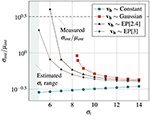Figure 7. σimtimt for the interspike interval plotted against σt for vgs = 1.8V with Constant, Gaussian, and Exponential Power (EP[κ], where κ is the shape factor) distributions of the threshold noise. The experimentally observed σimtimt for a VO2 neuron is shown with a dotted line. The shaded region shows the experimentally estimated range of σtt < 5).

## 4. Discussion

In this paper, we demonstrate and analyse an IMT based stochastic neuron hardware which relies on both threshold fluctuations and thermal noise as precursors to bifurcation. The IMT neuron emulates the functionality of theoretical neuron models completely by incorporating all neuron characteristics into device phenomena. Unlike other similar efforts, it does not need peripheral circuits alongside the core device circuit (an IMT device and a transistor) to emulate any sub-component of the spiking neuron model like thresholding, reset etc. Moreover, the neuron construction not only utilizes inherent physical noise sources for stochasticity, but also enables control of firing probability using an analog electrical signal—the gate voltage of series transistor. This is different from previous works which control only the deterministic aspect of firing rate like the charging rate. A comparison of spiking neuron hardware characteristics in different works is shown in Table 1.

TABLE 1Table 1. Comparison of this work (experimental details from Jerry et al., 2017a) with other spiking neuron hardware works based on different characteristics of spiking neurons.

We also show that the neuron dynamics follow a linear “carricature” of the FitzHugh-Nagumo model with intrinsic stochasticity. The analytical models developed in this paper can also faithfully reproduce the experimentally observed transfer curve which is a stochastic property. Such analytical verification of stochastic neuron experiments is one of the first in this work. It is an important result as it indicates reproducibility of stochastic characteristics and helps in creating the pathway toward perfecting these devices. With a growing concensus that stochasticity will play a key role in solving hard computing tasks, we need efficient ways for controlled amplification and conversion of physical noise into a readable and computable form. In this regard, the IMT based neuron represents a promising solution for a stochastic computational element. Such stochastic neurons have the potential to realize bio-mimetic computational kernels that can be employed to solve a large class of optimization and machine-learning problems.

## Author Contributions

AP worked on the development of theory, simulation frameworks, and mathematical models; MJ worked on the experiments; AR advised AP and participated in the problem formulation; SD advised MJ and also participated in the design of experiments and problem formulations.

## Conflict of Interest Statement

The authors declare that the research was conducted in the absence of any commercial or financial relationships that could be construed as a potential conflict of interest.

## Acknowledgments

This project was supported by the National Science Foundation under grants 1640081, Expeditions in Computing Award-1317560 and CCF- 1317373, and the Nanoelectronics Research Corporation (NERC), a wholly-owned subsidiary of the Semiconductor Research Corporation (SRC), through Extremely Energy Efficient Collective Electronics (EXCEL), an SRC-NRI Nanoelectronics Research Initiative under Research Task IDs 2698.001 and 2698.002.

## References

Buesing, L., Bill, J., Nessler, B., and Maass, W. (2011). Neural dynamics as sampling: a model for stochastic computation in recurrent networks of spiking neurons. PLoS Comput. Biol. 7:e1002211. doi: 10.1371/journal.pcbi.1002211

Cocker, T., Titova, L., Fourmaux, S., Holloway, G., Bandulet, H.-C., Brassard, D., et al. (2012). Phase diagram of the ultrafast photoinduced insulator-metal transition in vanadium dioxide. Phys. Rev. B 85:155120. doi: 10.1103/PhysRevB.85.155120

Gerstner, W., and Kistler, W. M. (2002). Spiking Neuron Models: Single Neurons, Populations, Plasticity. Cambridge: Cambridge university press.

Haykin, S. S. (2009). Neural Networks and Learning Machines. Prentice Hall.

Indiveri, G., Chicca, E., and Douglas, R. (2006). A VLSI array of low-power spiking neurons and bistable synapses with spike-timing dependent plasticity. IEEE Trans. Neural Netw. 17, 211–221. doi: 10.1109/TNN.2005.860850

Jerry, M., Parihar, A., Grisafe, B., Raychowdhury, A., and Datta, S. (2017a). “Ultra-low power probabilistic imt neurons for stochastic sampling machines,” in Proc. Symp. VLSI Technology (Kyoto), T186–T187.

Jerry, M., Parihar, A., Raychowdhury, A., and Datta, S. (2017b). “A random number generator based on insulator-to-metal electronic phase transitions,” in Device Research Conference (DRC), 2017 75th Annual (South Bend, IN), 1–2.

Jerry, M., Shukla, N., Paik, H., Schlom, D. G., and Datta, S. (2016). “Dynamics of electrically driven sub-nanosecond switching in vanadium dioxide,” in Silicon Nanoelectronics Workshop (SNW), 2016 IEEE (Honolulu, HI), 26–27.

Kar, A., Shukla, N., Freeman, E., Paik, H., Liu, H., Engel-Herbert, R., et al. (2013). Intrinsic electronic switching time in ultrathin epitaxial vanadium dioxide thin film. Appl. Phys. Lett. 102:072106. doi: 10.1063/1.4793537

McKean, H. P. (1970). Nagumo's equation. Adv. Math. 4, 209–223.

Mehonic, A., and Kenyon, A. J. (2016). Emulating the electrical activity of the neuron using a silicon oxide rram cell. Front. Neurosci. 10:57. doi: 10.3389/fnins.2016.00057

Parihar, A., Shukla, N., Datta, S., and Raychowdhury, A. (2015). Synchronization of pairwise-coupled, identical, relaxation oscillators based on metal-insulator phase transition devices: a model study. J. Appl. Phys. 117:054902. doi: 10.1063/1.4906783

Pickett, M. D., Medeiros-Ribeiro, G., and Williams, R. S. (2013). A scalable neuristor built with mott memristors. Nat. Mater. 12, 114–117. doi: 10.1038/nmat3510

Ricciardi, L. M., and Sato, S. (1988). First-passage-time density and moments of the ornstein-uhlenbeck process. J. Appl. Probab. 25, 43–57. doi: 10.2307/3214232

Sengupta, A., Panda, P., Wijesinghe, P., Kim, Y., and Roy, K. (2016). Magnetic tunnel junction mimics stochastic cortical spiking neurons. Sci. Rep. 6:30039. doi: 10.1038/srep30039

Shukla, N., Parihar, A., Cotter, M., Barth, M., Li, X., Chandramoorthy, N., et al. (2014a). “Pairwise coupled hybrid vanadium dioxide-MOSFET (HVFET) oscillators for non-boolean associative computing,” in 2014 IEEE International Electron Devices Meeting (San Francisco, CA), 28.7.1–28.7.4.

Shukla, N., Parihar, A., Freeman, E., Paik, H., Stone, G., Narayanan, V., et al. (2014b). Synchronized charge oscillations in correlated electron systems. Sci. Rep. 4:4964. doi: 10.1038/srep04964

Tashman, J., Lee, J., Paik, H., Moyer, J., Misra, R., Mundy, J., et al. (2014). Epitaxial growth of VO_2 by periodic annealing. Appl. Phys. Lett. 104:063104. doi: 10.1063/1.4864404

Tonnelier, A. (2003). The McKean's caricature of the Fitzhugh–Nagumo Model I. The space-clamped system. SIAM J. Appl. Math. 63, 459–484. doi: 10.1137/S0036139901393500

Tuma, T., Pantazi, A., Le Gallo, M., Sebastian, A., and Eleftheriou, E. (2016). Stochastic phase-change neurons. Nat. Nanotechnol. 11, 693–699. doi: 10.1038/nnano.2016.70

Zhang, K., Wang, B., Wang, F., Han, Y., Jian, X., Zhang, H., et al. (2016). VO2-based selection device for passive resistive random access memory application. IEEE Elec. Dev. Lett. 37, 978–981. doi: 10.1109/LED.2016.2582259

Keywords: stochastic neuron, insulator-metal transition, FitzHugh-Nagumo (FHN) neuron model, Ornstein-Uhlenbeck process, threshold noise, vanadium-dioxide

Citation: Parihar A, Jerry M, Datta S and Raychowdhury A (2018) Stochastic IMT (Insulator-Metal-Transition) Neurons: An Interplay of Thermal and Threshold Noise at Bifurcation. Front. Neurosci. 12:210. doi: 10.3389/fnins.2018.00210

Received: 25 August 2017; Accepted: 15 March 2018;
Published: 04 April 2018.

Edited by:

Themis Prodromakis, University of Southampton, United Kingdom

Reviewed by:

Adnan Mehonic, University College London, United Kingdom
Rune W. Berg, University of Copenhagen, Denmark

Copyright © 2018 Parihar, Jerry, Datta and Raychowdhury. This is an open-access article distributed under the terms of the Creative Commons Attribution License (CC BY). The use, distribution or reproduction in other forums is permitted, provided the original author(s) and the copyright owner are credited and that the original publication in this journal is cited, in accordance with accepted academic practice. No use, distribution or reproduction is permitted which does not comply with these terms.

*Correspondence: Abhinav Parihar, aparihar6@gatech.edu
Arijit Raychowdhury, arijit.raychowdhury@ece.gatech.edu